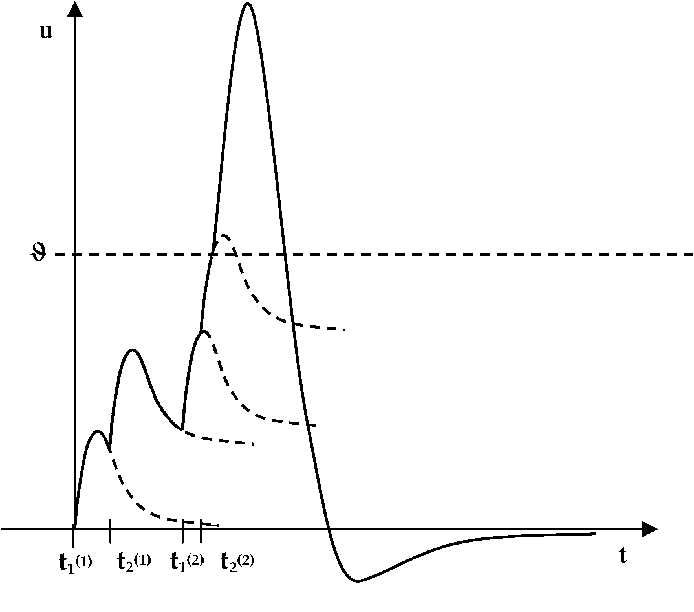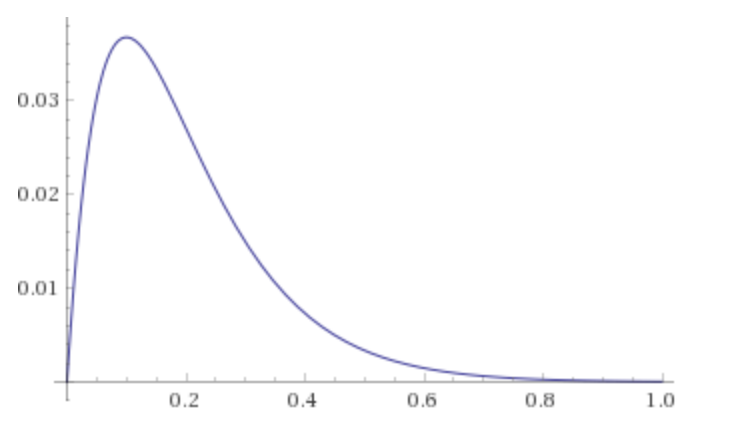# Temporal Coding in Spiking Neural Networks with Alpha Synaptic Function (Review)

## 1 Introduction

### 1.1 What are Spiking Neural Networks?

• NN architectures that mimics biological neural networks:

Motivation:

• low power consumption
• analog computation
• fast inference
• event-driven processing
• online learning
• parallelism

### 1.2 Spike Response Model (SRM) (Gerstner & Kistler, 2002)

Model for membrane potential:

\begin{equation} u_i(t) = \eta (t - \hat{t_i}) + \sum_{j}\sum_{f} \epsilon_{ij}(t - t_j^{(f)}) + u_{rest} \end{equation}

• Spikes come from the dendrites (input neurons), and this voltage accumulates
• Voltage decays slowly to resting potential
• Upon exceeding threshold, spike, and enter refractory period

### 1.3 Spiking, illustratedFigure 1: Membrane potential over time. Source: (Murilo Saraiva de Queiroz et al., 2006)

### 1.4 Spike Trains

• Sequence of (spike, timestamp)

### 1.5 How Do Neurons Encode Information?

1. Rate-coding

• Windowed average across:
• single neuron
• multiple runs
• population of neurons

Problems:

• Windowed average $$\rightarrow$$ minimum latency (brain has to wait for average)
• Research shows brain can act on single spikes
2. Temporal-coding

• Time to first spike
• Phase
• Correlations and Synchrony
3. Which coding scheme is better?

• Both codes are consistent: if the mean firing rate of a neuron is high, then the time to first spike is expected to occur early
• Rate codes discard temporal information

### 1.6 What’s the landscape for SNNs?

• Most SNNs cannot be trained with gradient-based methods, because there are no gradients
• The current approach to training SNNs include:
• Binarization of ANNs
• Conversion from ANNs
• Training of constrained networks
• Supervised learning with spikes
• Local learning rules at synapses
• Exception: probabilistic SNNs define outputs as jointly distributed random binary processes. The joint distributions are differentiable in the synaptic weights, and one can use principled learning criteria from ML and information theory

## 2 Temporal Coding using with Alpha Synaptic Function (Comsa et al., 2019)

### 2.1 Motivation

1. Atemporal networks (think LSTMs) don’t have the benefits of encoding information directly in the temporal domain
1. They remain sequential (require all previous layers of computation to produce answer)
2. Information in the real world are typically temporal

### 2.2 Key Ideas

1. Temporal Coding: Information is encoded in the relative timing of neuron spikes. Using temporal coding allows shift of differentiable relationship into the temporal domain.

1. Find differentiable relationship of the time of postsynaptic spike with respect to the weights and times of the presynaptic spikes.
2. Alpha synaptic transfer function: Use the SRM, but with the exponential decay of form $$t e^{-t}$$.

3. Synchronization pulses: input-independent spikes, used to facilitate transformations of the class boundaries.

### 2.3 The Coding Scheme

More salient information about a feature is encode as an earlier spike in the corresponding input neuron (think time-to-first-spike).

In a classification problem with $$m$$ inputs and $$n$$ possible classes:

input
spike times of $$m$$ input neurons
output
index of output neuron that fires first (among the $$n$$ output neurons)

### 2.4 Alpha Synaptic Function

Incoming exponential synaptic kernels are of the form $$\epsilon(t) = \tau^{-1}e^{-\tau t}$$ for some decay constant $$\tau$$. Potential of membrane in response to the spike is then $$u(t) = t e^{-\tau t}$$. It has a gradual rise, and slow decay.Figure 3: Plot of $$y = x e^{-10x}, x \in [0, 1]$$

### 2.5 Modelling Membrane Potential

The membrane potential is a weighted sum of the presynaptic inputs:

\begin{equation} V_{mem}(t) = \sum_{i} w_i (t-t_i)e^{\tau(t_i - t)} \end{equation}

We can compute the spike time $$t_{out}$$ of a neuron by considering the minimal subset of presynaptic inputs $$I_{t_{out}}$$ with $$t_i \le t_{out}$$ such that:

\begin{equation} \label{eqn:threshold} \sum_{i \in {I_{t_{out}}}} w_i \left( t_{out} - t_{i} \right) e^{\tau (t_i - t_{out})} = \theta \end{equation}

has 2 solutions: 1 on rising part of function and another on decaying part. The spike time is the earlier solution.

### 2.6 Solving for the Equation

Let $$A_{I} = \sum_{i \in I} w_i e^{\tau t_i}$$, and $$B_{I} = \sum_{i \in I} w_i e^{\tau t_i} t_i$$, we can compute:

\begin{equation} t_{out} = \frac{B_I}{A_I} - \frac{1}{\tau}W\left( -\tau \frac{\theta}{A_I}e^{\tau \frac{B_I}{A_I}} \right) \end{equation}

where $$W$$ is the Lambert W function.

### 2.7 The Loss Function

The loss minimizes the spike time of the target neuron, and maximizes the spike time of non-target neurons (cross-entropy!)

Softmax on the negative values of the spike times $$o_{i}$$ (which are always positive):

\begin{equation} p_j = \frac{e^{- o_j}}{\sum_{i=1}^{n} e^{- o_i}} \end{equation}

The cross entropy loss $$L(y_i, p_i) = - \sum_{i=1}^{n} y_i \ln p_i$$ is used.

Changing the weights of the network alters the spike times. We can compute the exact derivative of the post synaptic spike time wrt any presynaptic spike time $$t_j$$ and its weight $$w_j$$ as:

\begin{equation} \frac{\partial t_{out}}{\partial t_j} = \frac{w_j e^{t_j} \left( t_j - \frac{B_I}{A_I} + W_I + 1\right)}{A_I (1 + W_I)} \end{equation}

\begin{equation} \frac{\partial t_{out}}{\partial w_j} = \frac{e^{t_j} \left( t_j - \frac{B_I}{A_I} + W_I + 1\right)}{A_I (1 + W_I)} \end{equation}

where

\begin{equation} W_I = W\left( -\frac{\theta}{A_I}e^{\frac{B_I}{A_I}} \right) \end{equation}

### 2.8 Synchronization Pulses

These act as a temporal form of bias, adjusting class boundaries in the temporal domain. Per network, or per layer biases are added. Spike times for each pulse are learned with the rest of the parameters of the network.

## 3 Experiments

### 3.1 Boolean Logic Problems

Inputs encoded as individual spike times of two input neurons. All spikes occur between 0 and 1. True and False values are drawn from distributions $$[0.0, 0.45]$$ and $$[0.55, 1.0]$$ respectively.

Trained for maximum of 100 epochs, 1000 training examples. Tested on 150 randomly generated test examples. 100% accuracy on all problems.

### 3.2 Non-convolutional MNIST

784 neurons of the input layer corresponding to pixels of the image. Darker pixels encoded as earlier spike times. Output of network is the index of the earliest neuron to spike.

Trained with evolutionary-neural hybrid agents. Best networks achieved 99.96% and 97.96% accuracy on train and test sets.

The network learns two operating modes: slow-regime and fast-regime. Operating in the slow regime has higher accuracy, but takes more time. Fast regime makes quick decisions, with the first spike in the output layer occurring before the mean spike in the hidden layer.

## 4 Running the Code

### 4.1 Training the Model

Ignoring n_inputs flag for MNIST problem. Using 784 inputs.
Network architecture: [784, 340, 10]
Sync pulses: [0.0909091, 0.181818, 0.272727, 0.363636, 0.454545, 0.545455, 0.636364, 0.727273, 0.818182, 0.909091]
Saving the model that performs best on validation set.

run 0	epoch 0	train_error 0.97	train_acc.% 68.668519	valid acc.% 82.916664	elapsed 52207ms	Sync pulses: [[8.076, 8.23419, 0.189354, 0.338183, 8.56173, 1.18753, 0.407155, 9.37003, 1.0993, 9.06061], [9.09816, 2.53959, 9.10911, 9.25221, 0.243072, 9.12975, 8.56196, 4.01023, 9.1322, 5.54389], [0.0909091, 0.181818, 0.272727, 0.363636, 0.454545, 0.545455, 0.636364, 0.727273, 0.818182, 0.909091]]	LR: 0.00101864	batch sz: 32

run 0	epoch 1	train_error 0.38	train_acc.% 88.842593	valid acc.% 89.650002	elapsed 52526ms	Sync pulses: [[8.92665, 9.10365, 0, 0, 9.08495, 0, 2.74874, 10.427, 6.27137, 10.0179], [9.87262, 4.53841, 9.00944, 10], [0.0909091, 0.181818, 0.272727, 0.363636, 0.454545, 0.545455, 0.636364, 0.727273, 0.818182, 0.909091]]	LR: 0.00101864	batch sz: 32

run 0	epoch 2	train_error 0.29	train_acc.% 91.561111	valid acc.% 91.733330	elapsed 52074ms	Sync pulses: [[10.1715, 9.89629, 0.0372902, 0.0709029, 10.825, 0.105749, 3.72036, 11.2825, 7.87501, 11.2333], [10.3181, 5.71146, 10.3364, 11.5131, 2.54343, 11.4791, 10.4386, 3.77724, 11.4736, 8.09482], [0.0909091, 0.181818, 0.272727, 0.363636, 0.454545, 0.545455, 0.636364, 0.727273, 0.818182, 0.909091]]	LR: 0.00101864	batch sz: 32


### 4.2 Testing the Models

[nix-shell:~/projects/ihmehimmeli/build]\$ tempcoding/tempcoding_main -model_to_test=tempcoding/networks/slow_network -problem=mnist -n_test=10000 -n_train=60000 -n_validation=0 -decay_rate=0.181769 -mnist_data_path=../data/mnist
W2019-09-01T21:48:01.040269665+08:00 /home/jethro/projects/ihmehimmeli/tempcoding/spiking_problem.cc:417] Ignoring n_inputs flag for MNIST problem. Using 784 inputs.
IHM_CHECK(file.Open(path, mode)) failed at /home/jethro/projects/ihmehimmeli/tempcoding/file_passthrough_external.cc:97
Aborted


## 5 Thoughts

### 5.1 My Thoughts

Little information is lost with the temporal encoding scheme, so I’d expect the spiking neural network to perform well. Especially so, since there are gradients and gradient-based methods have already proven to be reliable.

Will augmenting gradients for a spiking neural network be useful in this scenario? Can we meta-learn for algorithms like STDP or equilibrium propagation instead?

# Bibliography

Gerstner, W., & Kistler, W. M., Spiking neuron models: single neurons, populations, plasticity (2002), : Cambridge university press.

Queiroz, M. S. d., Berrêdo, R. C. d., & Antônio de P\‘adua Braga, Reinforcement learning of a simple control task using the spike response model, Neurocomputing, 70(1-3), 14–20 (2006). http://dx.doi.org/10.1016/j.neucom.2006.07.002

Comsa, I. M., Potempa, K., Versari, L., Fischbacher, T., Gesmundo, A., & Alakuijala, J., Temporal coding in spiking neural networks with alpha synaptic function, CoRR, (), (2019).

Icon by Laymik from The Noun Project. Website built with ♥ with Org-mode, Hugo, and Netlify.